# 14.13: Organomagnesium and Organolithium Compounds in Synthesis

$$\newcommand{\vecs}{\overset { \rightharpoonup} {\mathbf{#1}} }$$ $$\newcommand{\vecd}{\overset{-\!-\!\rightharpoonup}{\vphantom{a}\smash {#1}}}$$$$\newcommand{\id}{\mathrm{id}}$$ $$\newcommand{\Span}{\mathrm{span}}$$ $$\newcommand{\kernel}{\mathrm{null}\,}$$ $$\newcommand{\range}{\mathrm{range}\,}$$ $$\newcommand{\RealPart}{\mathrm{Re}}$$ $$\newcommand{\ImaginaryPart}{\mathrm{Im}}$$ $$\newcommand{\Argument}{\mathrm{Arg}}$$ $$\newcommand{\norm}{\| #1 \|}$$ $$\newcommand{\inner}{\langle #1, #2 \rangle}$$ $$\newcommand{\Span}{\mathrm{span}}$$ $$\newcommand{\id}{\mathrm{id}}$$ $$\newcommand{\Span}{\mathrm{span}}$$ $$\newcommand{\kernel}{\mathrm{null}\,}$$ $$\newcommand{\range}{\mathrm{range}\,}$$ $$\newcommand{\RealPart}{\mathrm{Re}}$$ $$\newcommand{\ImaginaryPart}{\mathrm{Im}}$$ $$\newcommand{\Argument}{\mathrm{Arg}}$$ $$\newcommand{\norm}{\| #1 \|}$$ $$\newcommand{\inner}{\langle #1, #2 \rangle}$$ $$\newcommand{\Span}{\mathrm{span}}$$

## Additions to Carbonyl Groups. Synthesis of Alcohols

The most important synthetic use of Grignard reagents and organolithium reagents is to form new carbon-carbon bonds by addition to polar multiple bonds, particularly carbonyl bonds. An example is the addition of methyl-magnesium iodide to methanal:The yields of addition products in reactions of this kind are generally high. The adducts have metal-oxygen bonds that can be broken readily by acid hydrolysis to give the organic product. Grignard reagents seldom add to carbon-carbon multiple bonds (however, see Section 14-12D).

With suitable variations of the carbonyl compound, a wide range of compounds can be built up from substances containing fewer carbon atoms per molecule. The products formed when several types of carbonyl compounds react with Grignard reagents are listed in Table 14-4. The sequence of reactions starting with an organic halide, $$\ce{RX}$$, amounts to the addition of $$\ce{R-H}$$ across a carbonyl bond.$$^2$$Primary alcohols can be prepared by the addition of $$\ce{RMgX}$$ or $$\ce{RLi}$$ to methanal, $$\ce{CH_2=O}$$,Alcohols of formula $$\ce{RCH_2CH_2OH}$$ can be prepared by addition of $$\ce{RMgX}$$ to oxacyclopropane (oxirane):Table 14-4: Products from the Reaction of Grignard Reagents $$\left( \ce{RMgX} \right)$$ with Carbonyl Compounds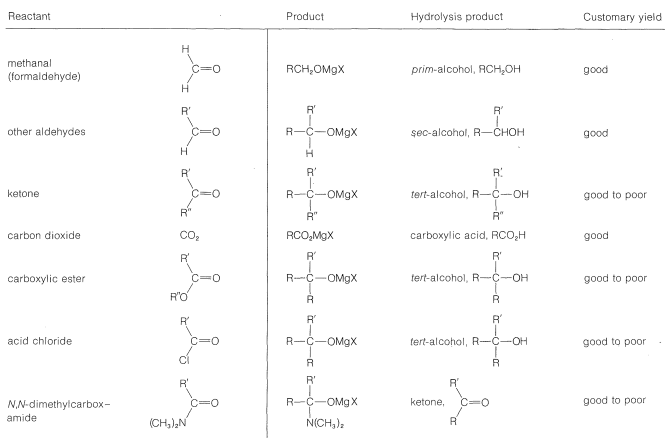Secondary alcohols are obtained from aldehydes, whereas ketones give tertiary alcohols: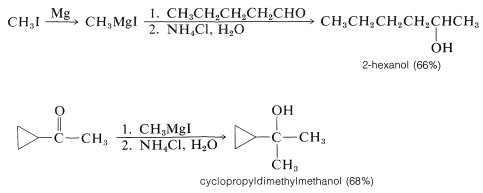Hydrolysis of the intermediate $$\ce{R-OMgX}$$ compound is achieved best with aqueous ammonium chloride solution. Addition of water gives an unpleasant mess of $$\ce{Mg(OH)_2}$$ whereas addition of strong acids such as $$\ce{HCl}$$ or $$\ce{H_2SO_4}$$ can lead to side reactions of dehydration and so on, especially with tertiary alcohols (Section 8-9C):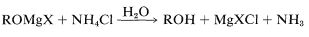Tertiary alcohols also are obtained from both acyl halides, $$\ce{RCOCl}$$, and esters, $$\ce{RCO_2R}$$, by the addition of two moles of Grignard reagent. The first mole of $$\ce{RMgX}$$ adds to the carbonyl bond to give the adducts $$13$$ or $$14$$: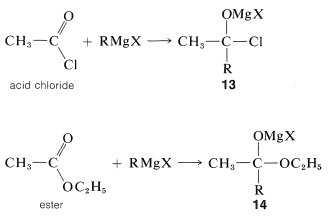However, these first-formed adducts are unstable and decompose to a ketone, $$\ce{CH_3COR}$$, and magnesium salts, $$\ce{MgXCl}$$ or $$\ce{MgXOC_2H_5}$$. The ketone usually cannot be isolated, but reacts rapidly with more $$\ce{RMgX}$$ ultimately to give a tertiary alcohol:Organolithium compounds behave very much like Grignard reagents, but with increased reactivity. They offer advantages over the magnesium compounds when the $$\ce{R}$$ group or the carbonyl compound is highly branched. For instance, isopropyllithium adds in good yield to 2,4-dimethyl-3-pentanone, whereas isopropylmagnesium bromide fails completely to give the normal addition product:Failure of Grignard reagents to add in the normal way generally is because reactions by alternative paths occur more rapidly. If the Grignard reagent has a hydrogen on the carbon adjacent to the point of attachment of $$\ce{-MgX}$$ (i.e., a $$\beta$$ hydrogen), then reduction can occur, with the effect of adding $$\ce{H_2}$$ to the carbonyl group.

Side reactions - reduction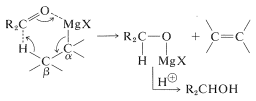Furthermore, if the carbonyl compound has a hydrogen located on the carbon next to the carbonyl group, the Grignard reagent can behave as a base and remove this hydrogen as a proton. The result is that the compound becomes an enolate salt and $$\ce{RMgX}$$ becomes $$\ce{RH}$$.

Side reactions - enolization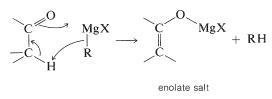Apparently, the complicating side reactions observed with $$\ce{RMgX}$$ are not nearly as important with $$\ce{RLi}$$. The reasons for this difference are not well understood.

## Synthesis of Carboxylic Acids

The reaction of carbon dioxide with Grignard reagents initially gives a magnesium salt of a carboxylic acid, $$\ce{RCO_2MgX}$$: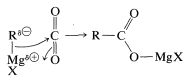This salt, which has a carbonyl group, in principle could add a second $$\ce{RMgX}$$. However, further addition is usually slow, and for most practical purposes the reaction stops at this stage. If the reaction can go further, the worst way to run it is by bubbling $$\ce{CO_2}$$ into the Grignard solution. This exposes the first-formed $$\ce{RCO_2MgX}$$ to excess $$\ce{RMgX}$$ and may lead to further addition reactions. The easy way to avoid this problem is to pour the $$\ce{RMgX}$$ solution onto powdered dry ice (solid $$\ce{CO_2}$$). Hydrolysis of the product (here a stronger acid than $$\ce{NH_4Cl}$$ is required) generates the carboxylic acid, $$\ce{RCO_2H}$$: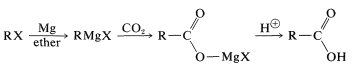## Synthesis of Ketones

Although organomagnesium compounds are not sufficiently reactive to add to carboxylate anions, alkyllithium compounds add quite well. A useful synthesis of methyl ketones involves the addition of methyllithium to the lithium salt of a carboxylic acid: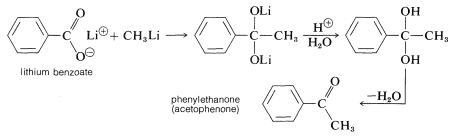Other methods begin with acid chlorides or esters and attempt to add only one mole of $$\ce{RMgX}$$: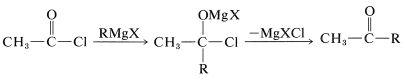The disadvantage of using Grignard reagents for this purpose is that they add very rapidly to the ketone as it is formed. There are two ways in which this disadvantage can be minimized. First, one can add the Grignard solution to an excess of acid chloride solution (the so-called “inverse addition” procedure) to keep the concentration of $$\ce{RMgX}$$ in the reaction mixture low, and hope that the reaction will stop at the ketone stage. However, this device seldom works very well with acid chlorides. Better results can be obtained with $$\ce{RMgX}$$ and $$\ce{R'CON(CH_3)_2}$$. The second method is to use a less reactive organometallic compound - one that will react with $$\ce{RCOCl}$$ but not with $$\ce{R_2C=O}$$. One easy way to do this is to add cadmium chloride to the Grignard solution, whereby an organocadmium compound, $$\ce{R_2Cd}$$, is formed (cf. Section 14-10B, Method 3). In the presence of magnesium halides, $$\ce{R_2Cd}$$ reacts moderately rapidly with acid chlorides, but only slowly with ketones. The addition therefore can be arrested at the ketone stage:

$2 \ce{RMgCl} + \ce{CdCl_2} \rightarrow \ce{R_2Cd} + 2 \ce{MgCl_2}$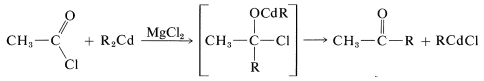Alkylcopper compounds, $$\ce{R-Cu}$$, also are selective reagents that react with acid chlorides to give ketones, but do not add to esters, acids, aldehydes, or ketones. The $$\ce{R-Cu}$$ compounds can be prepared from$$\ce{CuI}$$ and the alkyllithium. With an excess of the alkyllithium, the alkylcopper is converted to $$\ce{R_2CuLi}$$: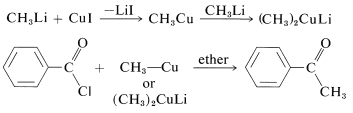## 1,4 Additions to Unsaturated Carbonyl Compounds

A conjugated alkenone,, can react with an organometallic reagent by a normal 1,2 addition across the carbonyl group, or by 1,4 addition to the conjugated system.On hydrolysis, the 1,4 adduct first yields the corresponding enol, but this is normally unstable and rearranges rapidly to the ketone. The final product therefore corresponds to addition of $$\ce{R-H}$$ across the carbon-carbon double bond:Organomagnesium and organolithium compounds can add both 1,2 and 1,4 to alkenones, and the relative importance of each mode of addition depends on the structure of the reactants. This sort of dual behavior can be a nuisance in synthetic work because it leads to separation problems and low yields. Organocopper compounds are a great help in this situation because they show a very high selectivity for 1,4 addition and add to unsaturated ketones in excellent yield:## Oxygen, Sulfur, and Halogens

Grignard reagents react with oxygen, sulfur, and halogens to form substances containing $$\ce{C-O}$$, $$\ce{C-S}$$, and $$\ce{C-X}$$ bonds, respectively:These reactions are not often important for synthesis because the products, $$\ce{ROH}$$, $$\ce{RSH}$$, and $$\ce{RX}$$, can be obtained more conveniently and directly from alkyl halides by $$S_\text{N}1$$ and $$S_\text{N}2$$ displacement reactions, as described in Chapter 8. However, when both $$S_\text{N}1$$ and $$S_\text{N}2$$ reactions are slow or otherwise impractical, as for neopentyl derivatives, the Grignard reactions can be very useful:Also, oxygenation of a Grignard reagent at low temperatures provides an excellent method for the synthesis of hydroperoxides:To prevent formation of excessive amounts of the alcohol, inverse addition is desirable (i.e., a solution of Grignard reagent is added to ether through which oxygen is bubbled rather than bubbling oxygen through a solution of the Grignard reagent).

Table 14-5: Methods of Preparation of Organic Halides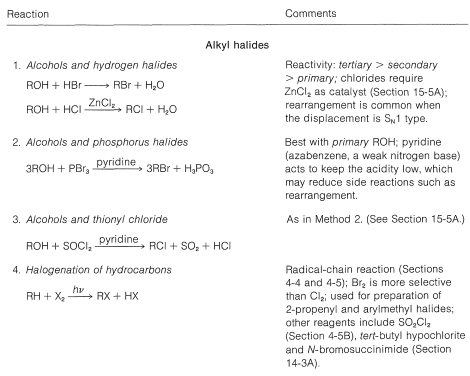Table 14-6: Reactivities of Organohalogen Compounds in Displacement and Elimination ReactionsTable 14-7: Methods of Preparation of Organometallic Compounds$$^2$$It is not possible to add $$\ce{RH}$$ to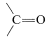directly because $$\Delta G^0$$ generally is somewhat unfavorable [$$+5 \: \text{kcal}$$ for $$\ce{CH_4} + \ce{(CH_3)_2C=O} \rightarrow \ce{(CH_3)_3COH}$$]. How we get around this unfavorable equilibrium in practice provides an interesting example of how energy can be (and is) squandered to achieve some particular desired result; for example, the reaction $$\ce{CH_3CH_3} + \ce{CH_3CHO} \rightarrow \ce{CH_3CH_3CH(CH_3)OH}$$ has $$\Delta H^0 = -12 \: \text{kcal}$$ but $$\Delta G^0 = + 0.5 \: \text{kcal}$$. A possible sequence is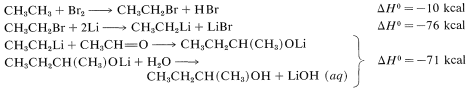The overall result is the expenditure of $$10 + 76 + 71 = 157 \: \text{kcal}$$ to achieve a reaction that itself has $$\Delta H^0 = -12 \: \text{kcal}$$, but an unfavorable $$\Delta G^0$$. ($$\ce{Li}$$ is used in this example rather than $$\ce{Mg}$$ because the heat of formation of $$\ce{C_2H_5MgBr}$$ is not available.)

## Contributors

John D. Robert and Marjorie C. Caserio (1977) Basic Principles of Organic Chemistry, second edition. W. A. Benjamin, Inc. , Menlo Park, CA. ISBN 0-8053-8329-8. This content is copyrighted under the following conditions, "You are granted permission for individual, educational, research and non-commercial reproduction, distribution, display and performance of this work in any format."